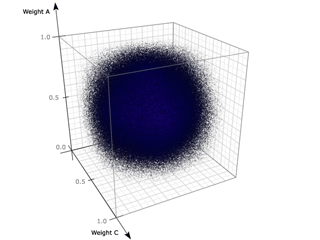# Appendix B. Advanced DataPoint Theory

 Warning Work-In-Progress This section is not yet finished.

Coming soon…

Weight Space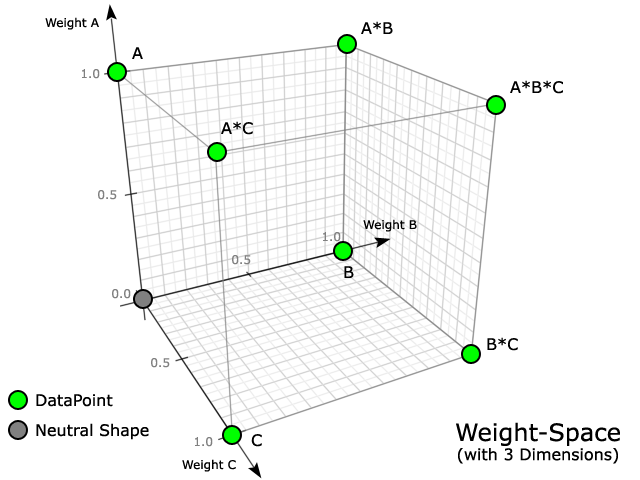DataPoint A (at position 1.0 of Weight A)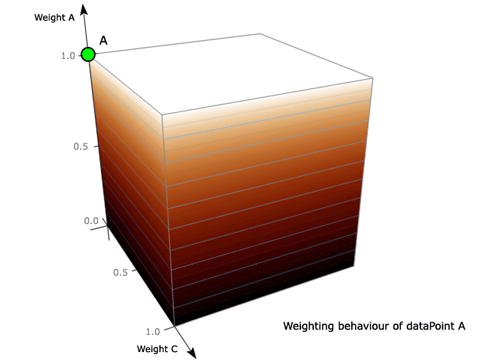Same DP cut in halfParticles showing DP weighting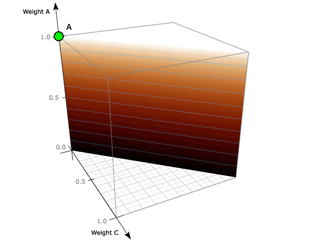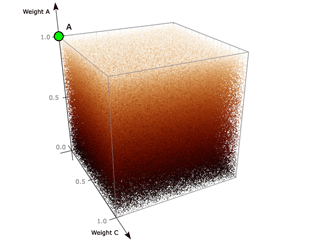DataPoint A*B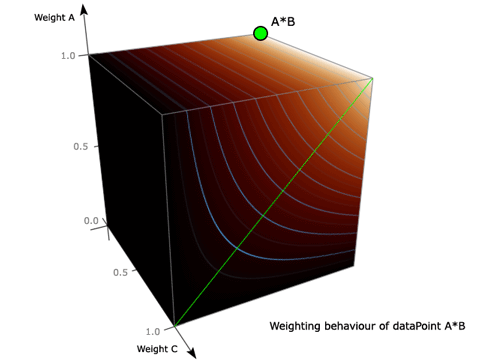Same DP cut in halfParticles showing DP weighting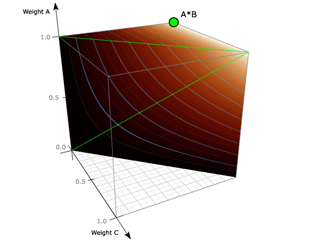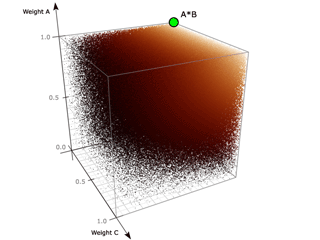DataPoint A*B*C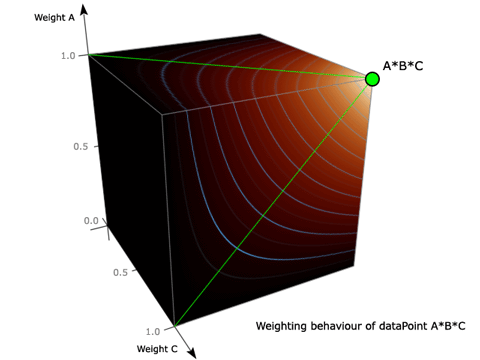Same DP cut in half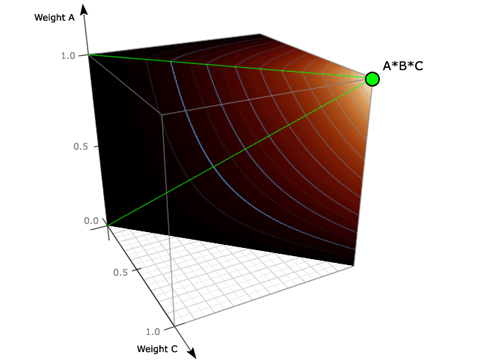Particles showing DP weighting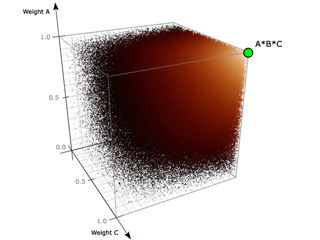Inbetween DataPoints in Weight Space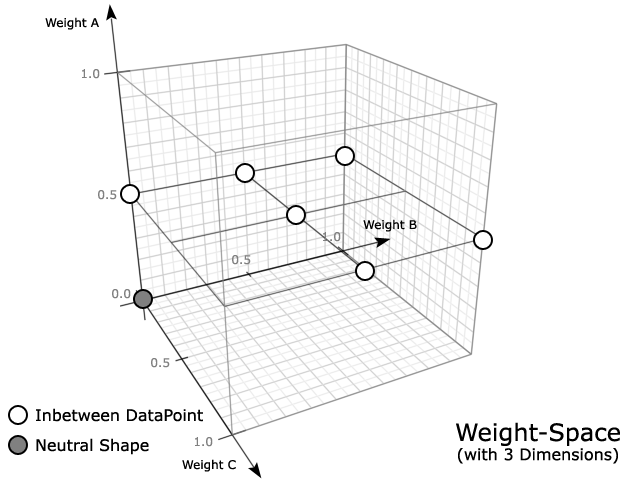### DataPoint at A(0.5)

Linear Interpolation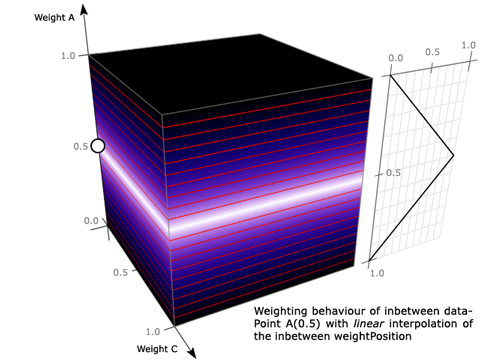Spline Interpolation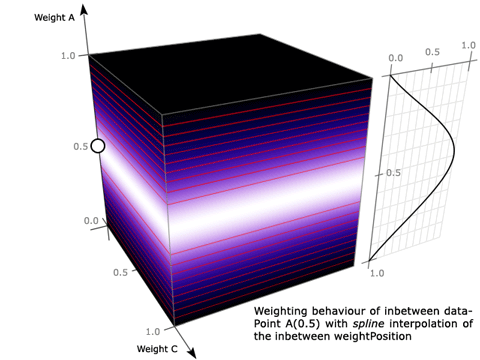Arc Interpolation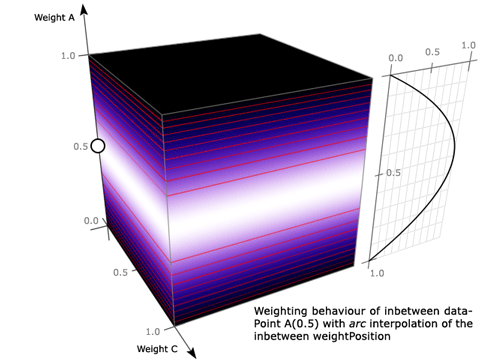Flat Interpolation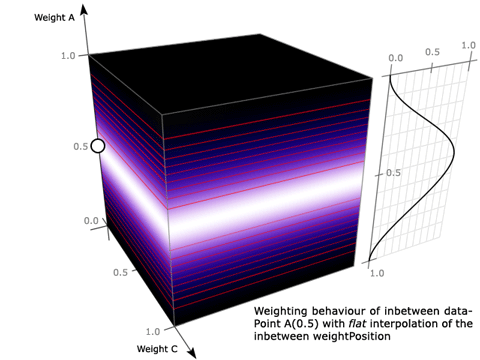Particles showing DP weighting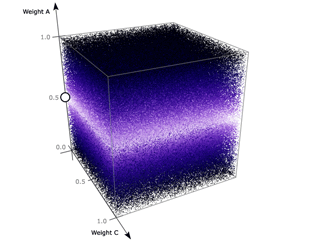### DataPoint at A(0.5)*B(1.0)

A(0.5)*B(1.0) with linear interpolation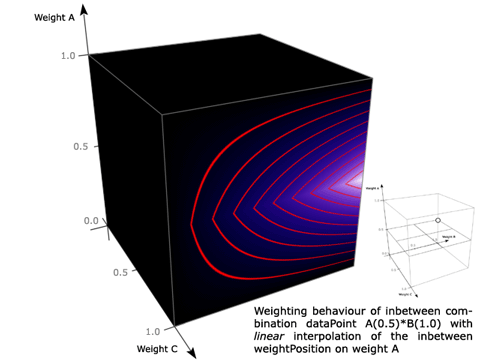Cut through the weight spaceParticles showing DP weighting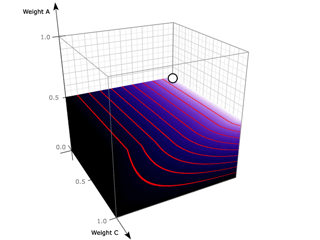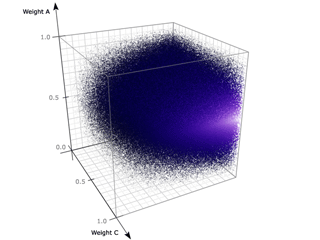Various weighting curves with linear interpolation on weight A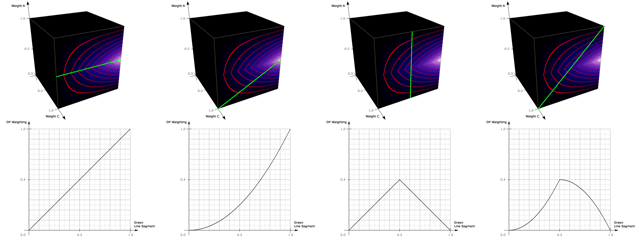Various weighting curves with spline interpolation on weight A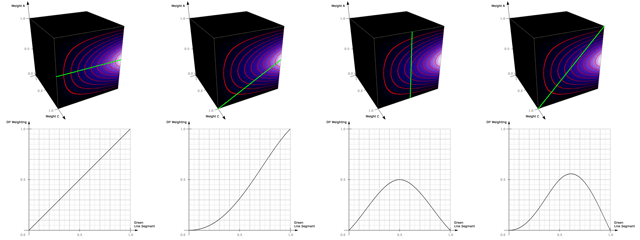### DataPoint at A(0.5)*B(0.5)

A(0.5)*B(0.5) with linear interpolation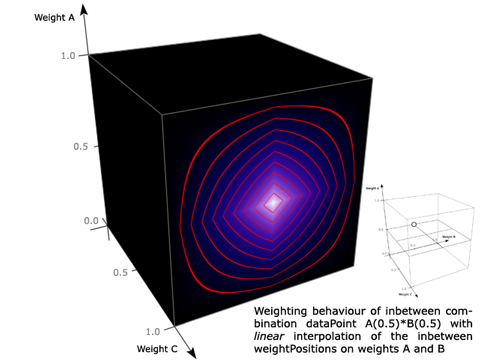Particles showing DP weighting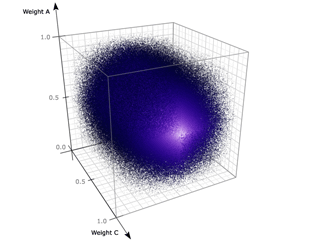LinearSpline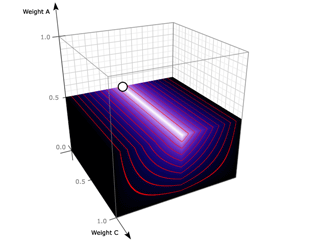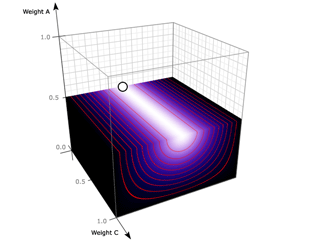ArcFlat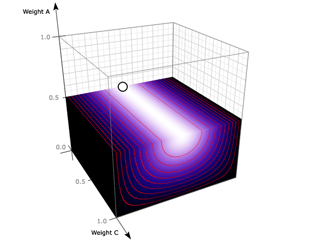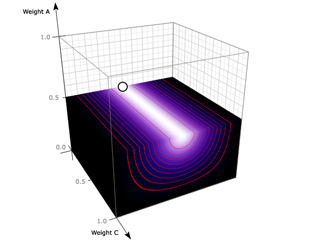### DataPoint at A(0.5)*B(1.0)*C(1.0)

A(0.5)*B(1.0)*C(1.0) with linear interpolation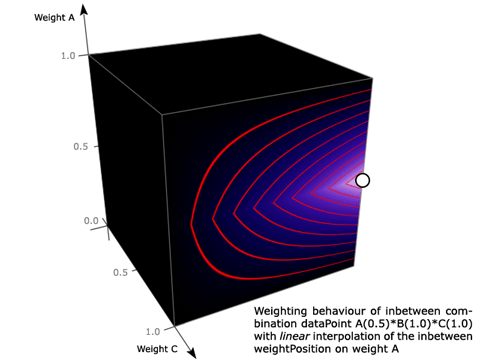Cut through the weight space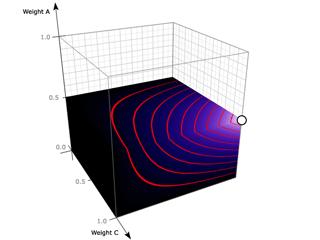### DataPoint at A(0.5)*B(0.5)*C(1.0)

A(0.5)*B(0.5)*C(1.0) with linear interpolation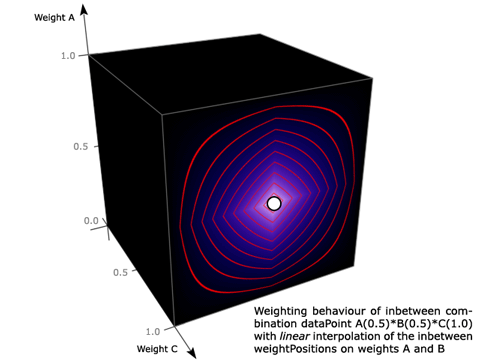Cut through the weight space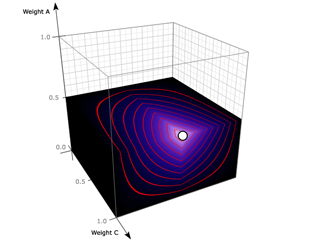### DataPoint at A(0.5)*B(0.5)*C(0.5)

A(0.5)*B(0.5)*C(0.5) with linear interpolation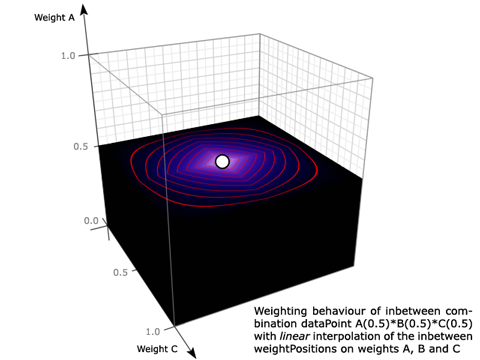Linear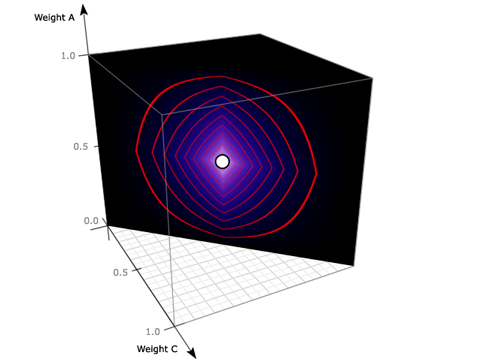Spline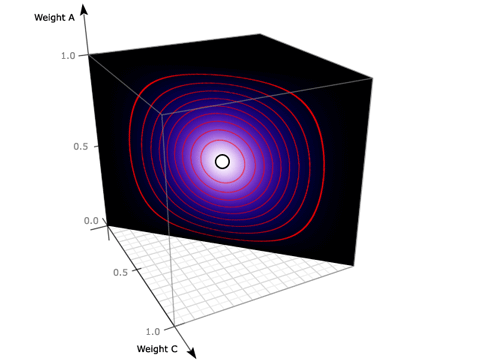Arc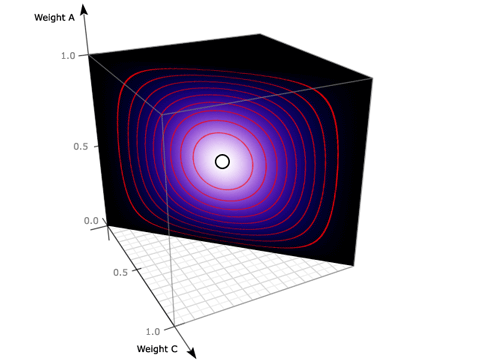Flat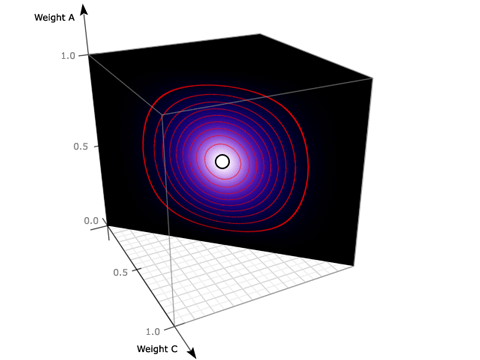Arc interpolation on weight A and flat on weights B and C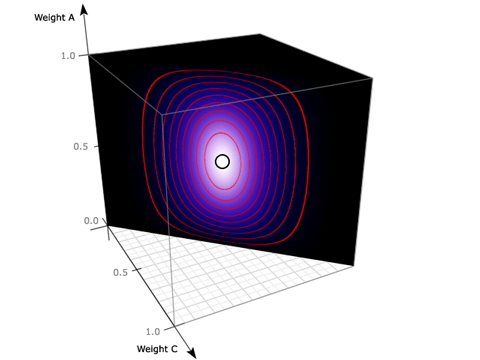Particles showing DP weighting